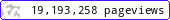•••••MadAsMaths.com :: I.Y.G.B. Practice Papers :: A Level Practice Papers 2019 Spec :: FP4 Practice Papers

I.Y.G.B. FP4 PRACTICE PAPERS

These I.Y.G.B. practice papers mostly follow the Pearson/Edexcel Syllabus introduced for teaching from 2017.

Some modules will be first examined in 2018 and some in 2019.

• Papers A to R have standard difficulty with later papers usually more difficult.
• Papers S and T are extremely hard.
• Papers U to Z are hard.

All papers have model solutions.

 fp4_a.pdffp4_a_solutions.pdffp4_b.pdffp4_b_solutions.pdffp4_c.pdffp4_c_solutions.pdffp4_d.pdffp4_d_solutions.pdffp4_e.pdffp4_e_solutions.pdffp4_f.pdffp4_f_solutions.pdffp4_g.pdffp4_g_solutions.pdffp4_h.pdffp4_h_solutions.pdffp4_i.pdffp4_i_solutions.pdffp4_j.pdffp4_j_solutions.pdffp4_k.pdffp4_k_solutions.pdffp4_l.pdffp4_l_solutions.pdffp4_m.pdffp4_m_solutions.pdffp4_n.pdffp4_n_solutions.pdffp4_o.pdffp4_o_solutions.pdffp4_p.pdffp4_p_solutions.pdffp4_q.pdffp4_q_solutions.pdffp4_r.pdffp4_r_solutions.pdffp4_s.pdffp4_s_solutions.pdffp4_t.pdffp4_t_solutions.pdffp4_u.pdffp4_u_solutions.pdffp4_v.pdffp4_v_solutions.pdffp4_w.pdffp4_w_solutions.pdffp4_x.pdffp4_x_solutions.pdffp4_y.pdffp4_y_solutions.pdffp4_z.pdffp4_z_solutions.pdf